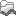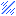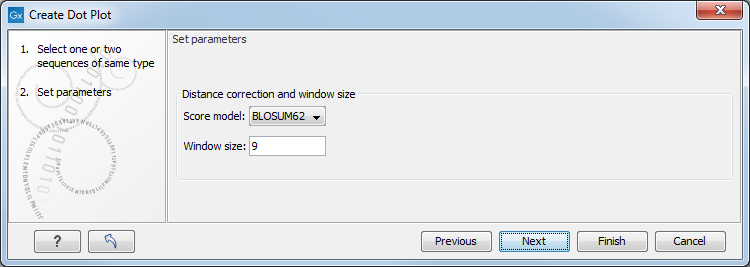## Create dot plots

To create a dot plot, go to:

Toolbox | Classical Sequence Analysis () | General Sequence Analysis ()| Create Dot Plot ()

In the dialog that opens, select a sequence and click Next to adjust dot plot parameters (figure 15.3).Figure 15.3: Setting the dot plot parameters.

There are two parameters for calculating the dot plot:

• Distance correction (only valid for protein sequences) In order to treat evolutionary transitions of amino acids, a distance correction measure can be used when calculating the dot plot. These distance correction matrices (substitution matrices) take into account the likeliness of one amino acid changing to another.
• Window size A residue by residue comparison (window size = 1) would undoubtedly result in a very noisy background due to a lot of similarities between the two sequences of interest. For DNA sequences the background noise will be even more dominant as a match between only four nucleotide is very likely to happen. Moreover, a residue by residue comparison (window size = 1) can be very time consuming and computationally demanding. Increasing the window size will make the dot plot more 'smooth'.

Note! Calculating dot plots takes up a considerable amount of memory in the computer. Therefore, you will see a warning message if the sum of the number of nucleotides/amino acids in the sequences is higher than 8000. If you insist on calculating a dot plot with more residues the Workbench may shut down, but still allowing you to save your work first. However, this depends on your computer's memory configuration.

Click Finish to start the tool.